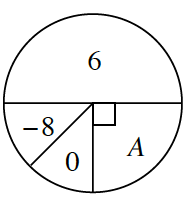Home > CCG > Chapter Ch7 > Lesson 7.3.1 > Problem7-121

7-121.Examine the spinner at right. Assume that the probability of spinning a $−8$ is equal to that of spinning a $0$.

1. Find the spinner’s expected value if the value of region $A$ is $8$.

Find the expected value of a single spin by finding the product of the probability and the value for each region. Find the sum for all the regions.

$P(6)·(6)+P(−8)·(−8)+P(0)·(0)+P(A)·(8)=\text{expected value}$

2. Find the spinner’s expected value if the value of region $A$ is $-4$.

Use the same equation from part (a), but put a $-4$ in place of the $8$ for the value of $A$.

$1$

3. What does the value of region $A$ need to be so that the expected value of the spinner is $0$?

Use the same equation from part (a), but put a $0$ in place of the $8$ for the value of $A$.

$-8$